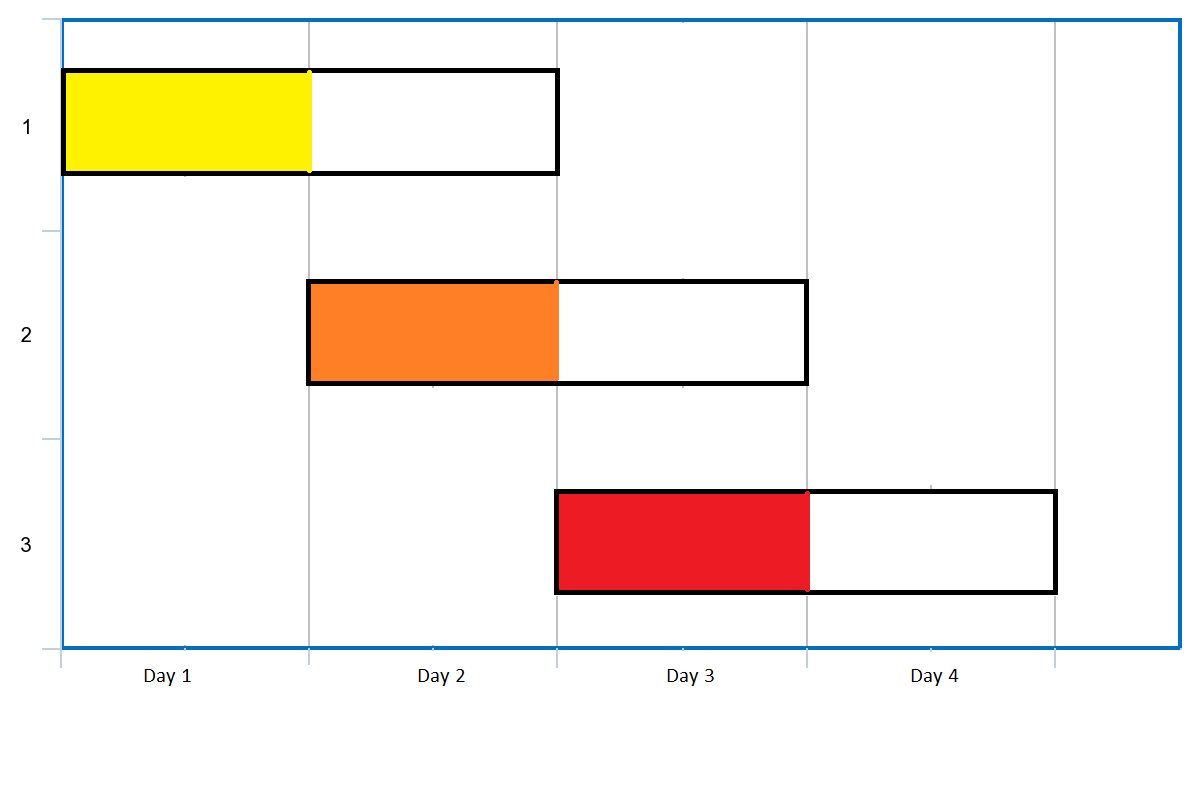##### Welcome to Subscribe On Youtube

Formatted question description: https://leetcode.ca/all/1353.html

# 1353. Maximum Number of Events That Can Be Attended (Medium)

Given an array of events where events[i] = [startDayi, endDayi]. Every event i starts at startDayi and ends at endDayi.

You can attend an event i at any day d where startTimei <= d <= endTimei. Notice that you can only attend one event at any time d.

Return the maximum number of events you can attend.

Example 1:Input: events = [[1,2],[2,3],[3,4]]
Output: 3
Explanation: You can attend all the three events.
One way to attend them all is as shown.
Attend the first event on day 1.
Attend the second event on day 2.
Attend the third event on day 3.


Example 2:

Input: events= [[1,2],[2,3],[3,4],[1,2]]
Output: 4


Example 3:

Input: events = [[1,4],[4,4],[2,2],[3,4],[1,1]]
Output: 4


Example 4:

Input: events = [[1,100000]]
Output: 1


Example 5:

Input: events = [[1,1],[1,2],[1,3],[1,4],[1,5],[1,6],[1,7]]
Output: 7


Constraints:

• 1 <= events.length <= 10^5
• events[i].length == 2
• 1 <= events[i] <= events[i] <= 10^5

Related Topics:
Greedy, Sort, Segment Tree

## Solution 1. Greedy

Sort the events in ascending order of the start time.

Let day be the current day and it starts with A.

If an event starts at day, we start to take it into consideration.

If an event ends before day, we can ignore it.

If an event starts no later than day and ends after day, we call it an active event.

Use a priority_queue to keep track of the end times of active events, and greedily pick the event with the earliest end time.

Here is a very good article explaining the reasoning step by step.

// OJ: https://leetcode.com/problems/maximum-number-of-events-that-can-be-attended/
// Time: O(NlogN)
// Space: O(N)
// Ref: https://leetcode.com/problems/maximum-number-of-events-that-can-be-attended/discuss/510263/JavaC%2B%2BPython-Priority-Queue
class Solution {
public:
int maxEvents(vector<vector<int>>& A) {
sort(A.begin(), A.end());
priority_queue<int, vector<int>, greater<int>> pq;
int N = A.size(), i = 0, day = A, ans = 0;
while (i < N || pq.size()) {
if (pq.empty()) day = A[i]; // If no active event is available and there are still more events to pick, jump to the start date of the next event.
while (i < N && A[i] == day) pq.push(A[i++]); // add events that start at day as active events, push their end time into queue
pq.pop(); // pick the event with the earliest start time
++ans;
++day;
while (pq.size() && pq.top() < day) pq.pop(); // ignore the events that are no longer active
}
return ans;
}
};

• class Solution {
public int maxEvents(int[][] events) {
PriorityQueue<int[]> priorityQueue = new PriorityQueue<int[]>(new Comparator<int[]>() {
public int compare(int[] event1, int[] event2) {
if (event1 != event2)
return event1 - event2;
else
return event1 - event2;
}
});
for (int[] event : events)
priorityQueue.offer(event);
int count = 0;
int curDay = 1;
while (!priorityQueue.isEmpty()) {
int[] event = priorityQueue.poll();
curDay = Math.max(curDay, event);
if (curDay <= event) {
curDay++;
count++;
}
while (!priorityQueue.isEmpty()) {
int[] nextEvent = priorityQueue.poll();
if (curDay <= nextEvent) {
priorityQueue.offer(nextEvent);
break;
}
if (curDay <= nextEvent) {
nextEvent = curDay;
priorityQueue.offer(nextEvent);
}
}
}
return count;
}
}

############

class Solution {
public int maxEvents(int[][] events) {
Map<Integer, List<Integer>> d = new HashMap<>();
int i = Integer.MAX_VALUE, j = 0;
for (var v : events) {
int s = v, e = v;
i = Math.min(i, s);
j = Math.max(j, e);
}
PriorityQueue<Integer> q = new PriorityQueue<>();
int ans = 0;
for (int s = i; s <= j; ++s) {
while (!q.isEmpty() && q.peek() < s) {
q.poll();
}
for (int e : d.getOrDefault(s, Collections.emptyList())) {
q.offer(e);
}
if (!q.isEmpty()) {
q.poll();
++ans;
}
}
return ans;
}
}

• class Solution:
def maxEvents(self, events: List[List[int]]) -> int:
d = defaultdict(list)
i, j = inf, 0
for s, e in events:
d[s].append(e)
i = min(i, s)
j = max(j, e)
h = []
ans = 0
for s in range(i, j + 1):
while h and h < s:
heappop(h)
for e in d[s]:
heappush(h, e)
if h:
ans += 1
heappop(h)
return ans


• func maxEvents(events [][]int) int {
d := map[int][]int{}
i, j := math.MaxInt32, 0
for _, v := range events {
s, e := v, v
d[s] = append(d[s], e)
i = min(i, s)
j = max(j, e)
}
q := hp{}
ans := 0
for s := i; s <= j; s++ {
for q.Len() > 0 && q.IntSlice < s {
heap.Pop(&q)
}
for _, e := range d[s] {
heap.Push(&q, e)
}
if q.Len() > 0 {
heap.Pop(&q)
ans++
}
}
return ans
}

func max(a, b int) int {
if a > b {
return a
}
return b
}

func min(a, b int) int {
if a < b {
return a
}
return b
}

type hp struct{ sort.IntSlice }

func (h *hp) Push(v interface{}) { h.IntSlice = append(h.IntSlice, v.(int)) }
func (h *hp) Pop() interface{} {
a := h.IntSlice
v := a[len(a)-1]
h.IntSlice = a[:len(a)-1]
return v
}
func (h *hp) Less(i, j int) bool { return h.IntSlice[i] < h.IntSlice[j] }